Difference between revisions of "User:IssaRice/Computability and logic/Eliezer Yudkowsky's Löb's theorem puzzle"

Translating the puzzle using logic notation

Löb's theorem shows that if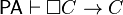$\mathsf{PA} \vdash \Box C \to C$, then$\mathsf{PA} \vdash C$.

The deduction theorem says that if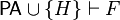$\mathsf{PA} \cup \{H\} \vdash F$, then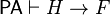$\mathsf{PA} \vdash H \to F$.

Applying the deduction theorem to Löb's theorem gives us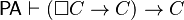$\mathsf{PA} \vdash (\Box C \to C) \to C$.

Translating the Löb's theorem back to logic

Since the solution to the puzzle refers back to the proof of Löb's theorem, we first translate the proof from the cartoon version back to logic:

1.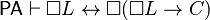$\mathsf{PA} \vdash \Box L \leftrightarrow \Box(\Box L \to C)$
2.$\mathsf{PA} \vdash \Box C \to C$
3.$\mathsf{PA} \vdash \Box(\Box L \to C) \to (\Box \Box L \to \Box C)$
4.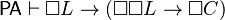$\mathsf{PA} \vdash \Box L \to (\Box \Box L \to \Box C)$
5.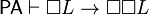$\mathsf{PA} \vdash \Box L \to \Box \Box L$
6.$\mathsf{PA} \vdash \Box L \to \Box C$
7.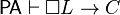$\mathsf{PA} \vdash \Box L \to C$
8.$\mathsf{PA} \vdash \Box(\Box L \to C)$
9.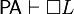$\mathsf{PA} \vdash \Box L$
10.$\mathsf{PA} \vdash C$